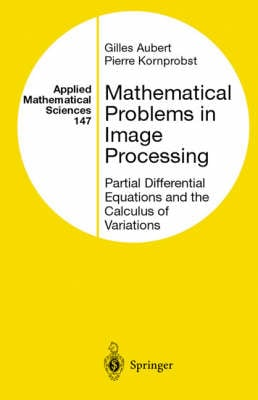Some orders may be delayed by the current Coronavirus lockdown.•# Mathematical Problems in Image Processing: v. 147: Partial Differential Equations and the Calculus of Variations - Applied Mathematical Sciences v.147 (Hardback)

(author), (author)
£48.50
Hardback 311 Pages / Published: 01/01/2002
• Not available

This product is currently unavailable

This product is currently unavailable.

Partial differential equations and variational methods were introduced into image processing about 15 years ago, and intensive research has been carried out since then. The main goal of this work is to present the variety of image analysis applications and the precise mathematics involved. It is intended for two audiences. The first is the mathematical community, to show the contribution of mathematics to this domain and to highlight some unresolved theoretical questions. The second is the computer vision community, to present a clear, self-contained, and global overview of the mathematics involved in image processing problems. The book is divided into five main parts. Chapter 1 is a detailed overview. Chapter 2 describes and illustrates most of the mathematical notions found throughout the work. Chapters 3 and 4 examine how PDEs and variational methods can be successfully applied in image restoration and segmentation processes. Chapter 5, which is more applied, describes some challenging computer vision problems, such as sequence analysis or classification. This book will be useful to researchers and graduate students in mathematics and computer vision.

Publisher: Springer-Verlag New York Inc.
ISBN: 9780387953267
Number of pages: 311
Weight: 590 g
Dimensions: 234 x 156 x 20 mm

MEDIA REVIEWS
From the reviews:

MATHEMATICAL REVIEWS

"The authors have substantially contributed to many aspects of the subjectThe level of the book is advanced, and the exposition very clear, developed in depth, and to some extent self-contained. The proofs of the main theorems are usually given in detail; when necessary there is also a precise reference to the ample literature on the specific topic. This book will certainly interest both mathematicians (some interesting, still open theoretical questions there are highlighted) and researchers in computer visionThe books substantial and up-to-date body for reference is a detailed guide to the literature on the many aspects of image processing."

G. Aubert and P. Kornprobst

Mathematical Problems in Image Processing

Partial Differential Equations and the Calculus of Variations

"This book is devoted to a detailed presentation of several aspects of mathematical problems in image processing . . . The authors have substantially contributed to many aspects of their subject . . . The level of the book is advanced, and the to some extent self-contained . . . This book will certainly interest both mathematicians (some interesting, still open theoretical questions here are highlighted) and researchers in computer vision."MATHEMATICAL REVIEWS

From the reviews:

MATHEMATICAL REVIEWS

"The authors have substantially contributed to many aspects of the subjecta ]The level of the book is advanced, and the exposition very clear, developed in depth, and to some extent self-contained. The proofs of the main theorems are usually given in detail; when necessary there is also a precise reference to the ample literature on the specific topic. This book will certainly interest both mathematicians (some interesting, still open theoretical questions there are highlighted) and researchers in computer visiona ]The books substantial and up-to-date body for reference is a detailed guide to the literature on the many aspects of image processing."

G. Aubert and P. Kornprobst

Mathematical Problems in Image Processing

Partial Differential Equations and the Calculus of Variations

"This book is devoted to a detailed presentation of several aspects of mathematical problems in image processing . . . The authors have substantially contributed to many aspects of their subject . . . The level of the book is advanced, and the to some extent self-contained . . . This book will certainly interest both mathematicians (some interesting, still open theoretical questions here are highlighted) and researchers in computer vision."a "MATHEMATICAL REVIEWS